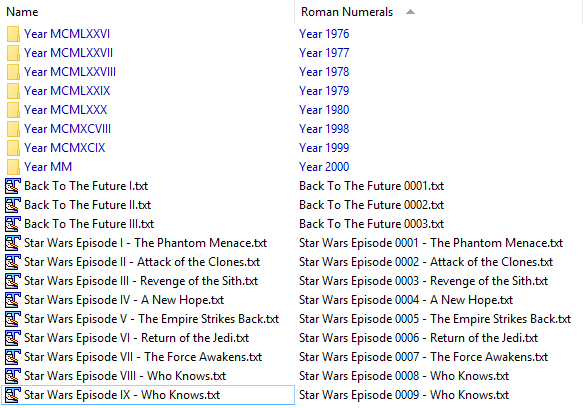# Column: Parse Roman Numerals for sorting names

### Overview:

This script adds a Roman Numerals column which shows the same as the Name column except that word in the name which is a valid roman numeral is converted into its corresponding decimal number, padded to 4 digits. You can thus sort by the column to sort things like titles and years which use roman numerals.Note: The script isn't magic. Words which a human being would not interpret as roman numerals, but which are technically valid ones, will still be converted. For example:

• `Me, Myself and I` converts to `My, Myself and 0001`

• `IV Drip.txt` converts to `0004 Drip.txt`

### Installation:

• Open Preferences / Toolbars / Scripts, then download Roman_Numerals_Column.js.txt (below) and drag it to the list.

• Roman_Numerals_Column.js.txt (1.45 KB)

### Usage:

If the script is installed, you should see its column under the Script category in the Folder Options dialog, or via the menu you get from right-clicking your existing columns:Add the columns manually as you would any others.

Alternatively, you might want to use a command, like this, which toggles between the column being hidden and the column being displayed & used to sort things:

``````@ifset:COLUMNSTOGGLE="scp:Roman Numerals Column/RomanNumerals"
Set COLUMNSREMOVE="scp:Roman Numerals Column/RomanNumerals"
@ifset:else
Set SORTBY="scp:Roman Numerals Column/RomanNumerals"
``````

### The script itself:

If you just want to use the script, the Roman_Numerals_Column.js.txt download above is probably easier.

The script code is reproduced here so that people looking for scripting techniques on the forum can browse the script code without having to open a separate file.

``````// Roman Numerals Column

function OnInit(initData)
{
initData.name = "Roman Numerals Column";
initData.desc = "A column showing file names with roman numerals converted to numbers";
initData.version = "1.0";
initData.default_enable = true;

col.name = "RomanNumerals";
col.method = "OnRomanNumerals";
col.label = "Roman Numerals";
col.justify = "left";
col.autogroup = true;
col.namerefresh = true;
col.autorefresh = false;
}

// Implement the RomanNumerals column
function OnRomanNumerals(scriptColData)
{
if (scriptColData.col != "RomanNumerals")
return;

scriptColData.value = deromWords(scriptColData.item.name_stem) + scriptColData.item.ext;
}

function deromWords(str) {
var out = "";
var num;
var words = str.split(" ");
for (var widx in words)
{
if (widx > 0)
out += " ";
num = deromanize(words[widx]);
if (num == 0)
out += words[widx];
else
out += num;
}
return out;
}

function deromanize(str)
{
var str = str.toUpperCase(),
validator = /^M*(?:D?C{0,3}|C[MD])(?:L?X{0,3}|X[CL])(?:V?I{0,3}|I[XV])\$/,
token = /[MDLV]|C[MD]?|X[CL]?|I[XV]?/g,
key = {M:1000,CM:900,D:500,CD:400,C:100,XC:90,L:50,XL:40,X:10,IX:9,V:5,IV:4,I:1},
num = 0, m;
if (!(str && validator.test(str)))
return 0;
while (m = token.exec(str))
num += key[m];
if (num > 0)
{
num = String(num);
while (num.length < 4)
num = "0" + num;
}
return num;
}
``````

Parts of the deromanize function were taken from here:

http://blog.stevenlevithan.com/archives/javascript-roman-numeral-converter